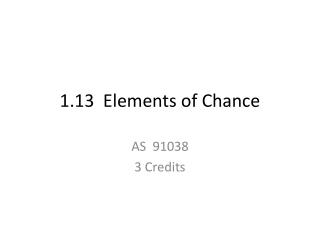DownloadDownload Presentation1.13 Elements of Chance

# 1.13 Elements of Chance

Download Presentation## 1.13 Elements of Chance

- - - - - - - - - - - - - - - - - - - - - - - - - - - E N D - - - - - - - - - - - - - - - - - - - - - - - - - - -
##### Presentation Transcript

1. 1.13 Elements of Chance AS 91038 3 Credits

2. aim of this unit: You need to investigate a situation involving elements of chance. ie you’re going to look at an experiment and comment on it, well more of write a repot on it.

3. Achievement • You need to investigate a situation involving elements of chance. • This involves showing evidence of using the experimental probability process. • You need to be familiar with: • posing a question to explore a situation (see Simulation) involving elements of chance • planning an experiment to explore the situation • discussing and defining the set of possible outcomes • deciding the sample size • gathering data by performing the experiment • selecting and using appropriate displays including experimental probability distributions • identifying and communicating patterns in the data • comparing discrete theoretical distributions and experimental distributions as appropriate • communicating findings in a conclusion.

4. Achievement with merit Make sure that you can meet the criteria for achievement. You need to investigate, with justification, a situation involving elements of chance. This involves linking aspects of the investigation to the situation, and making supporting statements which refer to evidence such as summary statistics, probabilities, trends or features of visual displays.

5. Achievement with excellence Make sure that you can meet the criteria for merit You need to investigate, showing statistical insight, a situation involving elements of chance. This involves integrating contextual information and knowledge with an understanding of applications of probability and may involve considering the possible effects of other related variables or factors.

6. Subject content • Know how to: • pose a question to explore a situation involving elements of chance • plan an experiment to explore the situation • discussing and defining the set of possible outcomes • deciding the sample size • gather data by performing the experiment • select and use appropriate displays including experimental probability distributions • identify and communicate patterns in the data • compare discrete theoretical distributions and experimental distributions as appropriate • communicate findings in a conclusion. • Visit these pages: • Probability • Simulation • Revise bar graphs, histograms and pie charts: • Displaying data • Statistical Graphs, Charts and Plots • Histograms vs. Bar Graphs • What is the difference between a bar graph and a histogram? • Simulation • Pie Graphs

7. Stuff to Practice before you try an experiment Probability Simple probability and experimental probability S-cool – Probability Theoretical Versus Empirical Probability Revision and practise on probability Revision Bite - probability (visit all three pages) Test Bite – probability Sample Space Practice with Sample Spaces Tree diagrams Tree Diagrams Working with Tree Diagrams S-cool: Tree diagrams Revision Bite – Probability - higher (visit all three pages) Conditional probability Revision Bite – Conditional probabilities S-cool – Rules of Probability

8. Simulation To describe a simulation use words and show these steps: Explain what tool you are going to use. Define what represents a successful trial. Give a table of the outcomes of several trials. Show how to calculate required probabilities. Interactive examples of simulation Chances Are Casio – worksheets: See Statistical Simulations, Statistical Simulations 2, Statistical Simulations 3. Queues (See student and teacher notes under this heading.) Let’s Make a Deal The Birthday Problem: A short lesson in probability Cliff-Hanger CensusAtSchool

9. Key tips Be familiar with outcomes based on using dice, coins, and playing cards. Practise using simulations to solve probability problems. Record your data carefully. Check you have addressed every requirement that was asked for in planning your investigation. Use appropriate graphs to display your data. They should enable you to show features in relation to your investigation. Summarise your findings, referring to the steps you took to come to your conclusion. You may be expected to complete probability trees and use these. Multiply the probabilities along the branches of tree diagrams if you are asked for the probability that an event AND another event occur. Add the probabilities at the end of the branches if you are asked for the probability that an event OR another event occurs. Probabilities are best written as fractions, but may be written as a decimal or percentage. If written as a percentage, you must include the percentage sign. Probability must not be written as a ratio.

10. All this information came from Studyit The exercises in the Nulake book support this work.